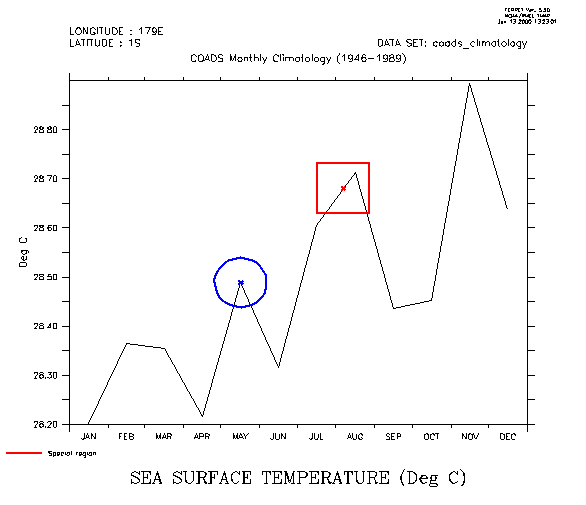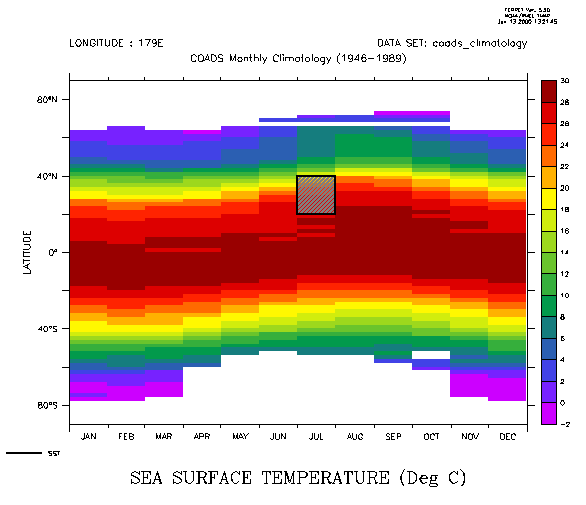# Overlaying on a time-axis plot.

## Questions:

```
How can I do overlays on a plot with a formatted time axis?

Explanation:
To overlay symbols or mark-up on a plot which has a formatted  time axis
(dates and times) it is necessary to specify  positions using the internal
time encoding of that axis.  Typically, the easiest way to achieve this
is to define a  variable, say TT, which is the time encoding. This example
illustrates.  These examples are taken from the Ferret Users Guide, Chapter 6.

Example:

demonstrate PLOT/VS and POLYGON over time plotLET xsqr = {-1,1,1,-1}                ! coordinates of a unit square
LET ysqr = {-1,-1,1,1}

LET xcircle = COS(6.3*i[i=1:42]/40)    ! coordinates of unit circle
LET ycircle = SIN(6.3*i[i=1:42]/40)

!   Notice the units of the time axis
show grid/l=1:3 sst

plot/x=180/y=0 sst     !  draw a  time series plot

let tt = T[gt=sst]         ! tt is the coordinates along the T axis

!   place an "X" at the value exactly at 7-aug
!   "@ITP" causes interpolation to exact location

let t0   = tt[t="7-aug-0000"@itp]
let val0 = sst[x=180,y=0,t="7-aug-0000"@itp]
plot/vs/over/nolab/line=8/color=red t0,val0

!   put a box around the "X"
polygon/over/line=8/title="Special region" t0+500*xsqr, 0.05*ysqr+val0

!   place an "X" on the data point nearest to 15-may
!   Note that @ITP is absent, so behavior is set by MODE INTERPOLATE

let t1   = tt[t="15-may-0000"]
let val1 = sst[x=180,y=0,t="15-may-0000"]
plot/vs/over/nolab/sym=2/line=10 t1,val1

!     put a circle around the "X"
plot/vs/over/line=10/nolab t1+500*xcircle,0.05*ycircle+val1

Example (continued):

mark-up over a Hofmuller (time vs space) diagramshade/x=180 sst                             !  latitude vs time plot
let tlo = tt[t="1-jul-0000"@itp]
let thi = tt[t="1-aug-0000"@itp]
polygon/over/line=7/pal=gray/pat=lite_up_left_to_right {`tlo`, `thi`,` thi`, `tlo`},  {20, 20, 40, 40}